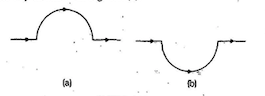Home/Class 12/Physics/

A straight wire carrying a current of  $$12\;A$$  is bent into a semi-circular arc of radius  $$2.0\;\mathit{cm}$$  as shown in  $$\mathit{Fig.}(a).$$  Consider the magnetic field  $$B$$  at the Centre of the arc. Would your answer be different if the wire were bent into a semi-circular arc of the same radius but in the opposite way as shown in $$\mathit{Fig.}(b).$$?Speed
00:00
01:14## QuestionPhysicsClass 12

A straight wire carrying a current of  $$12\;A$$  is bent into a semi-circular arc of radius  $$2.0\;\mathit{cm}$$  as shown in  $$\mathit{Fig.}(a).$$  Consider the magnetic field  $$B$$  at the Centre of the arc. Would your answer be different if the wire were bent into a semi-circular arc of the same radius but in the opposite way as shown in $$\mathit{Fig.}(b).$$?See the analysis below
4.64.6## Solution

Since the radius of both the circular arc is same, the magnitude of the magnetic field will be same. But as the direction of current is different in both the circular arc, the direction of the magnetic field will be different.